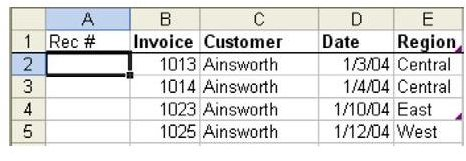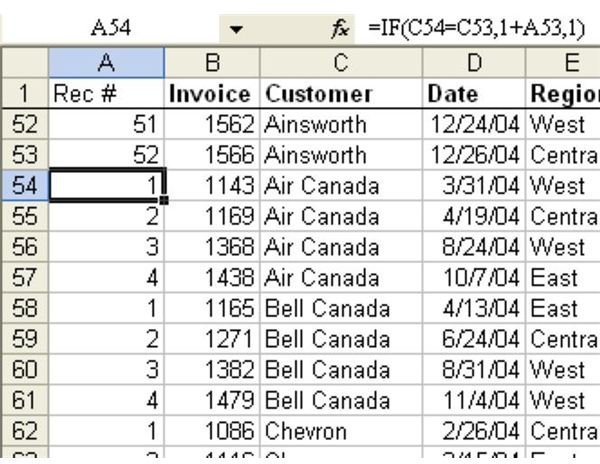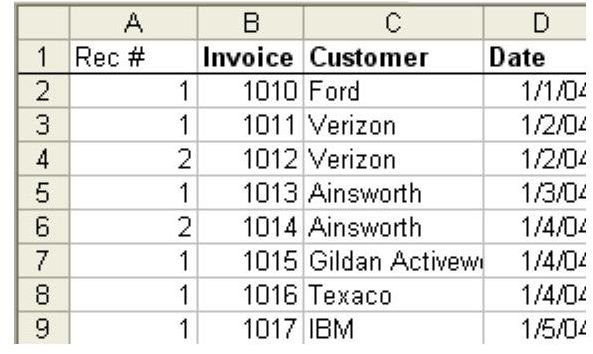# Learn How To Number Each Record For A Customer, Starting At One For A New Customer With This Microsoft Excel Tutorial

Page content

Strategy: Sort the data by Customer. Insert a new temporary column A and add a heading called “Rec #”, as shown in Fig. 811.

The formula in A2 is =IF(C2=C1,1+A1,1). In plain language, this formula says, “If the customer in C is equal to the customer above me, then add 1 to the cell above me. Otherwise, start at 1.”

Result: Each group of customer invoices is numbered from 1 to N, as shown in Fig. 812.

As shown in Fig. 813, copy the formulas in column A and Paste Special – Values to change to numbers so that you can re-sort the data by Invoice Number.

Alternate Strategy: A formula of =COUNTIF(\$C\$2:C2,C2) will work without sorting.

Summary: The IF function is perfect for this task of comparing the current record to the record above.

Functions Discussed:

=IF()

See all Microsoft Excel tips

## Images